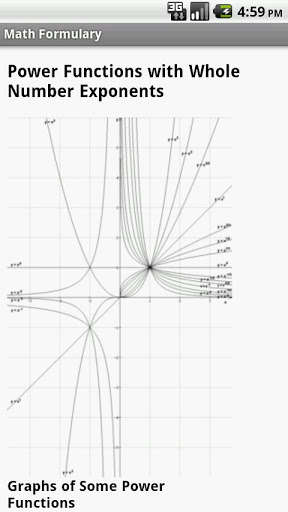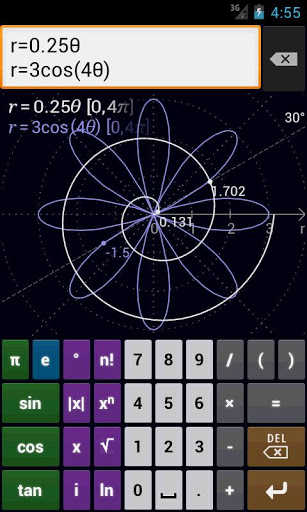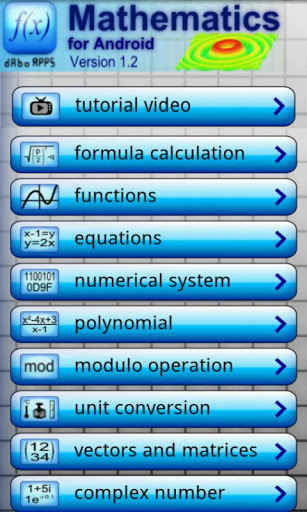There comes a time in every child’s life when he must face a mighty foe, or rather a few foes, one bigger and scarier than the other. This trio of horrors are: math, physics and chemistry. These three courses have made the lives of thousands of kids very hard, and although I do not approve of this, there are many apps targeted to help kids and students with their calculations.

As a student I find these math, physics and chemistry apps very useful, helping make complex calculations that would require some time to make, very easy and time effective. From my experience, they help you the most in exams, when time is limited and you have a huge workload to do.

### Top Android Math Apps

Because math is one of the hardest subjects you will attend, and because for many students, the time wasted on doing lots of calculations can be put to more use, the Play Market offers quite a lot of math apps, capable of resolving equations and other complex calculations. Here is our top of some of the best.

#### 7. MathStudioMathStudio is probably the most complete Android math app on the Play Store. It can do all the calculation you can think of, it can handle all the problems you throw at it and it’s very simple to use. Why is it the bottom ranking app in our top? Because it costs over \$10, while the other apps featured here are free. If this is not an inconvenience for you, than MathStudio will be the best app.

#### 6. Math RefFeaturing over 1400 equations, examples and tips for solving problems, this app can be regarded as a portable math teacher. It gives you all the information you need, very neatly structured and explained so you can learn all the math theories without the need for thousands of pages of text or hundreds of manuals. Give it a try and see how well it performs and how good the information it provides is.

#### 5. Math Algebra Solver CalculatorOne of the most simple apps in this top is Math Algebra Solver Calculator. This app is targeted at students who want to quickly solve math problems and get directly to the answer. For some, this might not be a good app because it does not show you the entire solving process, but nevertheless, a good app to check your results and be assured that you have solved your problems correctly.

#### 4. MathwayFor those who have used math resolving software and websites in the past, Mathway is a familiar name that needs no introduction. This service allows you to quickly resolve math problems in no time, and not, you can benefit from this service on your Android device. This free app give you all the tools you need to resolve any mathematical equation, no matter how complex.

#### 3. Math FormularyAlthough this app is not a calculator, and more of an learning app, Math Formulary gives a complete encyclopedia of mathematical knowledge. You can see definitions and explanations of any mathematical formula and examples for each of them so you can better understand how they work. A great tool for students all over the world because, they say, math is a universal language.

#### 2. Mathlab Graphing CalculatorIf you are looking for an Android math app that can both solve equations and render graphs, then the Mathlab Graphing Calculator is what you need. A simple, yet effective app that can resolve pretty much any equation you throw at it. This is a must have app for any engineering student who wants all the features of other, more powerful programs in one simple and free app.

#### 1. MathematicsI found this app to give almost all the utilities you could ever need in your math courses. It can help you create complex 3D graphs and solve equations, matrices complex number calculations and many more. If you are a student looking for a good math calculator, look no further, this is the app you need.

Now you have all the tools you need for both learning math and for doing calculations. These apps are targeted more for students, so they can quickly go through the calculations and concentrate on other tasks. So, you can see that Google offers yet another way to help students. Keep it up! Also, stay tuned, as we will look at other science apps, both for Android and iOS.